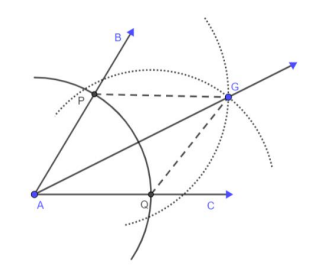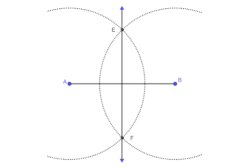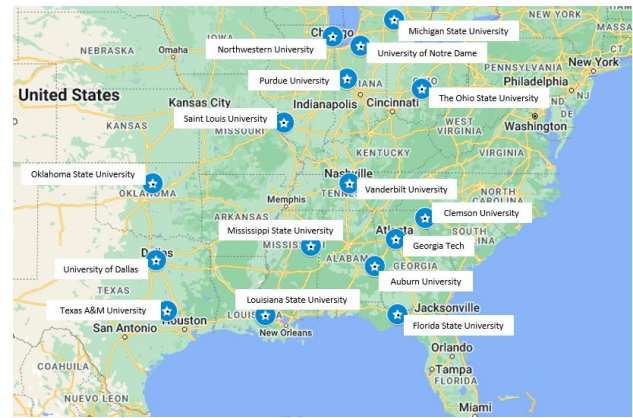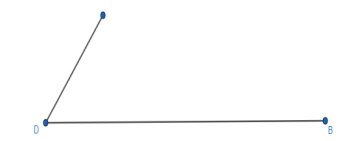# MA.912.GR.5.2Export Print
Construct the bisector of a segment or an angle, including the perpendicular bisector of a line segment.

### Clarifications

Clarification 1: Instruction includes using compass and straightedge, string, reflective devices, paper folding or dynamic geometric software.
General Information
Subject Area: Mathematics (B.E.S.T.)
Strand: Geometric Reasoning
Status: State Board Approved

## Benchmark Instructional Guide

• Angle

### Vertical Alignment

Previous Benchmarks

Next Benchmarks

### Purpose and Instructional Strategies

In elementary grades, students drew lines and angles using a variety of tools, including rulers and protractors, and by making measurements with those tools, they could bisect lines and angles. In Geometry, students are introduced to constructions that do not rely on making measurements, specifically bisecting an angle and bisecting a segment, including perpendicular bisectors, using a compass and straightedge. These two procedures are embedded within constructing an inscribed circle and a circumscribed circle of a triangle as well as in the construction of a square inscribed in a circle.
• Instruction includes the use of manipulatives, tools and geometric software. Allowing students to explore constructions with dynamic software reinforces why the constructions work.
• For example, the use of paper folding (e.g., patty paper) can be used to determine the angle bisector of a given angle and the midpoint or perpendicular bisector of a given segment.
• Instruction includes the connection to triangle congruence when constructing an angle bisector.
• For example, have students place the compass at point A and draw an arc intersecting the sides of the angle resulting in the points of intersection P and Q. Students should realize that APAQ. Without changing the compass setting, add two arcs intersecting in the interior of the angle at the point G. Students should realize that PGQG By the Reflexive property of congruence,  AGAG Therefore, ΔAPG ≅ ΔAQG by SSS and since corresponding parts of congruent triangles are congruent (CPCTC), ∠PAG ≅ ∠QAG and AG is the angle bisector of ∠BAC• Instruction includes the connection to the converse of the Perpendicular Bisector Theorem when constructing a perpendicular bisector.
• For example, students can set the compass width more than half the length of AB. Students can draw arcs intersecting above and below the segment at points E and F. Therefore, AEBE and  AF ≅  BF. That is, points E and F are each the same distance to the endpoints of AB and that means they lie on the Perpendicular Bisector.• Instruction includes the student understanding that when one has constructed the perpendicular bisector, they have also constructed the midpoint of a segment. (MTR.2.1
• For example, using the same steps as in the last construction, the midpoint of the segment can be identified as the point where the perpendicular bisector meets the segment.
• Instruction includes the connection to logical reasoning and visual proofs when verifying that a construction works.
• For example, once the construction of the perpendicular bisector is completed, discuss with students how this construction and a compass can be used to experimentally check the Perpendicular Bisector Theorem. (MA.912.GR.1.1
• For expectations of this benchmark, constructions should be reasonably accurate and the emphasis is to make connections between the construction steps and the definitions, properties and theorems supporting them.
• While going over the steps of geometric constructions, ensure that students develop vocabulary to describe the steps precisely. (MTR.4.1
• Instruction includes the connection between constructions and properties of quadrilaterals, including rhombi.
• For example, when constructing the angle bisector, if the compass width is not changed throughout the process, then quadrilateral AHGF is a rhombus since it has 4 equal sides ( AH HG, GF FA). The diagonals of a rhombus bisect opposite angles. Therefore, ∠HAF is bisected by the diagonal of the rhombus AG and AG is the angle bisector of ∠BAC. Similarly, when constructing the perpendicular bisector, it can be seen that the diagonals of a rhombus are perpendicular.
• Instruction includes the student understanding that in a geometric construction, one does not use the markings on a ruler or on a protractor to bisect a segment or angle. Students should realize that there are limitations on precision that are inherent in the markings on rulers or protractors.
• It is important to build the understanding that formal constructions are valid when the lengths of segments or measures of angles are not known, or have values that do not appear on a ruler or protractor, including irrational values.
• Problem types include identifying the next step of a construction, a missing step in a construction or the order of the steps in a construction.

### Common Misconceptions or Errors

• Students may not make the connection that any point on the perpendicular bisector is equidistant from the endpoints of the segment, not just the midpoint of the segment.
• Students may not understand why they are not using rulers and protractors to bisect segments and angles.

• A map of some popular universities is shown below.• Part A. Prove that Georgia Tech is approximately equidistant from Clemson University and Auburn University.
• Part B. Find one or more universities that are approximately equidistant from Florida State University and Oklahoma State University?

### Instructional Items

Instructional Item 1
• An image is provided below.• Part A. Construct the bisector of angle D
• Part B. Construct the midpoint of segment DB.

*The strategies, tasks and items included in the B1G-M are examples and should not be considered comprehensive.

## Related Courses

This benchmark is part of these courses.
1200400: Foundational Skills in Mathematics 9-12 (Specifically in versions: 2014 - 2015, 2015 - 2022, 2022 and beyond (current))
1206310: Geometry (Specifically in versions: 2014 - 2015, 2015 - 2022, 2022 and beyond (current))
1206320: Geometry Honors (Specifically in versions: 2014 - 2015, 2015 - 2022, 2022 and beyond (current))
1206315: Geometry for Credit Recovery (Specifically in versions: 2014 - 2015, 2015 - 2022, 2022 and beyond (current))
7912065: Access Geometry (Specifically in versions: 2015 - 2022, 2022 and beyond (current))

## Related Access Points

Alternate version of this benchmark for students with significant cognitive disabilities.
MA.912.GR.5.AP.2: Construct the bisector of a segment, including the perpendicular bisector of a line segment.

## Related Resources

Vetted resources educators can use to teach the concepts and skills in this benchmark.

## Formative Assessments

Bisecting a Segment and an Angle :

Students are asked to construct the bisectors of a given segment and a given angle and to justify one of the steps in each construction.

Type: Formative Assessment

Constructions for Perpendicular Lines:

Students are asked to construct a line perpendicular to given line (1) through a point not on the line and (2) through a point on the line.

Type: Formative Assessment

## Lesson Plans

Keeping Triangles in Balance: Discovering Triangle Centroid is Concurrent Medians:

In this lesson, students identify, analyze, and understand the Triangle Centroid Theorem. Students discover that the centroid is the point of concurrency for the medians of a triangle and recognize its associated usage with the center of gravity or barycenter. This set of instructional materials provides the teacher with hands-on activities using technology as well as paper-and-pencil methods.

Type: Lesson Plan

What's the Point? Part 2:

In this lesson, students use a paper-folding technique to discover the properties of angle bisectors. At the conclusion of the activity, students will be able to compare/contrast the points of concurrency of perpendicular and angle bisectors.

Type: Lesson Plan

Circumnavigating the Circumcenter:

Students use the concurrent point of perpendicular bisectors of triangle sides to determine the circumcenter of three points. Students will reason that the circumcenter of the vertices of a polygon is the optimal location for placement of a facility to service all of the needs of sites at the vertices forming the polygon.

Type: Lesson Plan

Right turn, Clyde!:

Students will develop their knowledge of perpendicular bisectors & point of concurrency of a triangle, as well as construct perpendicular bisectors through real world problem solving with a map.

Type: Lesson Plan

Halfway to the Middle!:

Students will develop their knowledge of mid-segments of a triangle, construct and provide lengths of mid-segments.

Type: Lesson Plan

Location, Location, Location, Location?:

Students will use their knowledge of graphing concurrent segments in triangles to locate and identify which points of concurrency are associated by location with cities and counties within the Texas Triangle Mega-region.

Type: Lesson Plan

St. Pi Day construction with a compass & ruler:

St. Pi Day construction with compass

This activity uses a compass and straight-edge(ruler) to construct a design. The design is then used to complete a worksheet involving perimeter, circumference, area and dimensional changes which affect the scale factor ratio.

Type: Lesson Plan

What's the Point? Part 1:

This is a patty paper-folding activity where students measure and discover the properties of the point of concurrency of the perpendicular bisectors of the sides of a triangle.

Type: Lesson Plan

## Original Student Tutorials

Angle UP: Player 1:

Explore the construction processes for constructing an angle bisector, copying an angle and constructing a line parallel to a given line through a point not on the line using a variety of tools in this interactive, retro video game-themed tutorial.

NOTE: This tutorial uses both the angle bisector construction and the construction to copy an angle as an extension opportunity to also construct a line parallel to a given line through a point not on the line. Students also learn to identify corresponding angles created when a transversal crosses parallel lines, and discover using Geogebra that these angles are congruent.

Type: Original Student Tutorial

Meet Me Half Way:

Plan a paddle board expedition by learning how to do basic geometric constructions including copying a segment, constructing a segment bisector, constructing a segment's perpendicular bisector and constructing perpendicular segments using a variety of tools in this interactive tutorial.

Type: Original Student Tutorial

The Blueprints of Construction:

Learn to construct the perpendicular bisector of a line segment using a straightedge and compass with this interactive tutorial.

Type: Original Student Tutorial

## MFAS Formative Assessments

Bisecting a Segment and an Angle :

Students are asked to construct the bisectors of a given segment and a given angle and to justify one of the steps in each construction.

Constructions for Perpendicular Lines:

Students are asked to construct a line perpendicular to given line (1) through a point not on the line and (2) through a point on the line.

## Original Student Tutorials Mathematics - Grades 9-12

Angle UP: Player 1:

Explore the construction processes for constructing an angle bisector, copying an angle and constructing a line parallel to a given line through a point not on the line using a variety of tools in this interactive, retro video game-themed tutorial.

NOTE: This tutorial uses both the angle bisector construction and the construction to copy an angle as an extension opportunity to also construct a line parallel to a given line through a point not on the line. Students also learn to identify corresponding angles created when a transversal crosses parallel lines, and discover using Geogebra that these angles are congruent.

Meet Me Half Way:

Plan a paddle board expedition by learning how to do basic geometric constructions including copying a segment, constructing a segment bisector, constructing a segment's perpendicular bisector and constructing perpendicular segments using a variety of tools in this interactive tutorial.

The Blueprints of Construction:

Learn to construct the perpendicular bisector of a line segment using a straightedge and compass with this interactive tutorial.

## Student Resources

Vetted resources students can use to learn the concepts and skills in this benchmark.

## Original Student Tutorials

Angle UP: Player 1:

Explore the construction processes for constructing an angle bisector, copying an angle and constructing a line parallel to a given line through a point not on the line using a variety of tools in this interactive, retro video game-themed tutorial.

NOTE: This tutorial uses both the angle bisector construction and the construction to copy an angle as an extension opportunity to also construct a line parallel to a given line through a point not on the line. Students also learn to identify corresponding angles created when a transversal crosses parallel lines, and discover using Geogebra that these angles are congruent.

Type: Original Student Tutorial

Meet Me Half Way:

Plan a paddle board expedition by learning how to do basic geometric constructions including copying a segment, constructing a segment bisector, constructing a segment's perpendicular bisector and constructing perpendicular segments using a variety of tools in this interactive tutorial.

Type: Original Student Tutorial

The Blueprints of Construction:

Learn to construct the perpendicular bisector of a line segment using a straightedge and compass with this interactive tutorial.

Type: Original Student Tutorial

## Parent Resources

Vetted resources caregivers can use to help students learn the concepts and skills in this benchmark.